# How to Calculate and Solve for Actual Mass of Geological Body | Gravity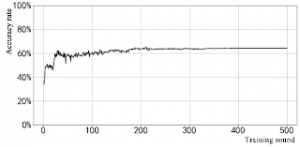The image above represents actual mass of geological body.

To compute for actual mass of geological body three essential parameters are needed and these parameters are Total Anomalous Mass (ME), Anomalous Density of the Body (ρ1and Host Rock Density (ρ0).

The formula for calculating actual mass of geological body:

M = ME (ρ1 / ρ1 – ρ0)

Where:

M = Actual Mass of Geological Body
ME = Total Anomalous Mass
ρ1 = Anomalous Density of the Body
ρ0 = Host Rock Density

Let’s solve an example;
Find the actual mass of geological body when the total anomalous mass is 12, anomalous density of the body is 21 and the host rock density is 13.

This implies that;

ME = Total Anomalous Mass = 12
ρ1 = Anomalous Density of the Body = 21
ρ0 = Host Rock Density = 13

M = ME (ρ1 / ρ1 – ρ0)
M = 12 (21 / 21 – 13)
M = 12 (21 / 8)
M = 12 (2.625)
M = 31.5

Therefore, the actual mass of geological body is 31.5 kg.

Calculating the Total Anomalous Mass when the Actual Mass of Geological Body, the Anomalous Density of the Body and the Host rock Density is Given.

ME = M / (ρ1 / ρ1 – ρ0)

Where;

ME = Total Anomalous Mass
M = Actual Mass of Geological Body
ρ1 = Anomalous Density of the Body
ρ0 = Host Rock Density

Let’s solve an example;
Find the total anomalous mass when the actual mass of geological body is 45, the anomalous density of the body is 12 and the host rock density is 10.

This implies that;

M = Actual Mass of Geological Body = 45
ρ1 = Anomalous Density of the Body = 12
ρ0 = Host Rock Density = 10

ME = M / (ρ1 / ρ1 – ρ0)
ME = 45 / (12 / 12 – 10)
ME = 45 / (12 / 2)
ME = 45 / 6
ME = 7.5

Therefore, the actual mass of geological body is 7.5 kg.

Nickzom Calculator – The Calculator Encyclopedia is capable of calculating the actual mass of geological body.

To get the answer and workings of the actual mass of geological body using the Nickzom Calculator – The Calculator Encyclopedia. First, you need to obtain the app.

You can get this app via any of these means:

To get access to the professional version via web, you need to register and subscribe for NGN 2,000 per annum to have utter access to all functionalities.
You can also try the demo version via https://www.nickzom.org/calculator

Android (Paid) – https://play.google.com/store/apps/details?id=org.nickzom.nickzomcalculator
Android (Free) – https://play.google.com/store/apps/details?id=com.nickzom.nickzomcalculator
Apple (Paid) – https://itunes.apple.com/us/app/nickzom-calculator/id1331162702?mt=8
Once, you have obtained the calculator encyclopedia app, proceed to the Calculator Map, then click on Geology under Add-on.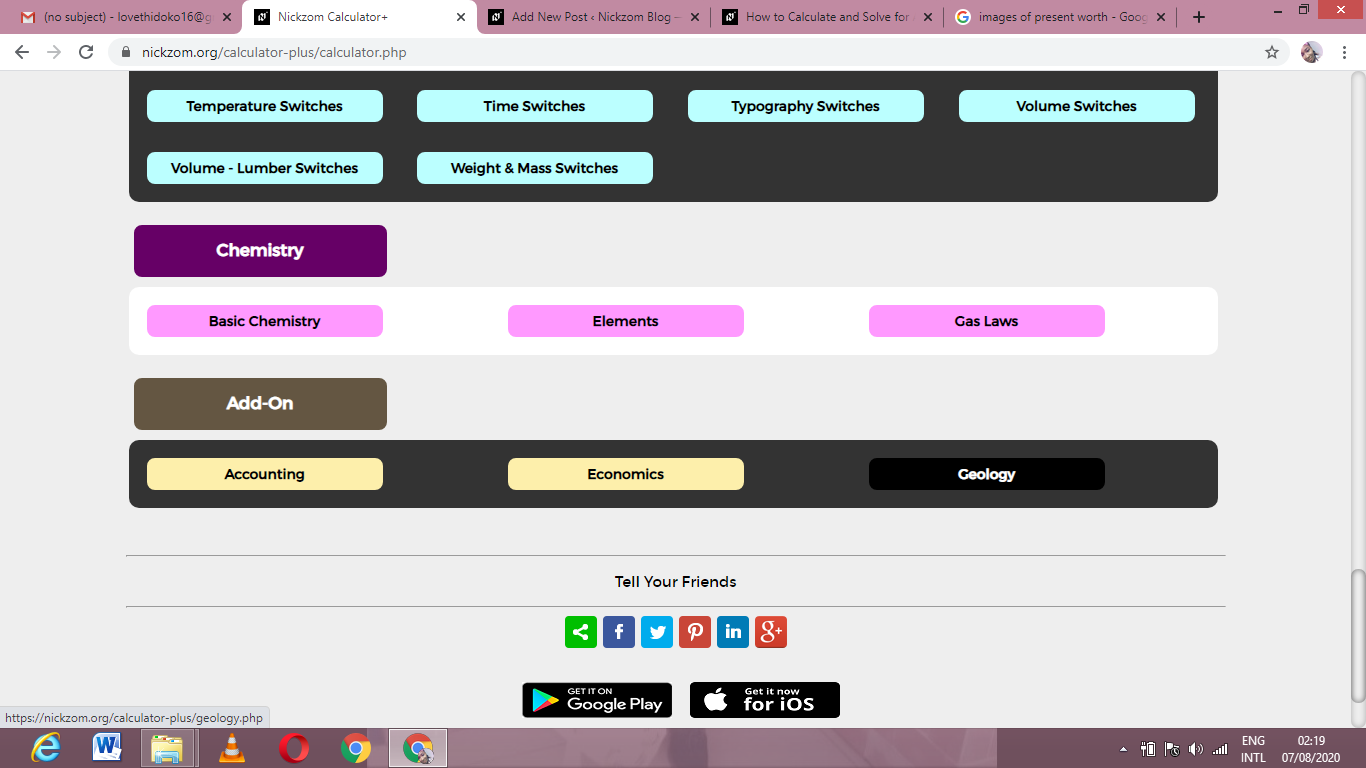Now, Click on Gravity under Geology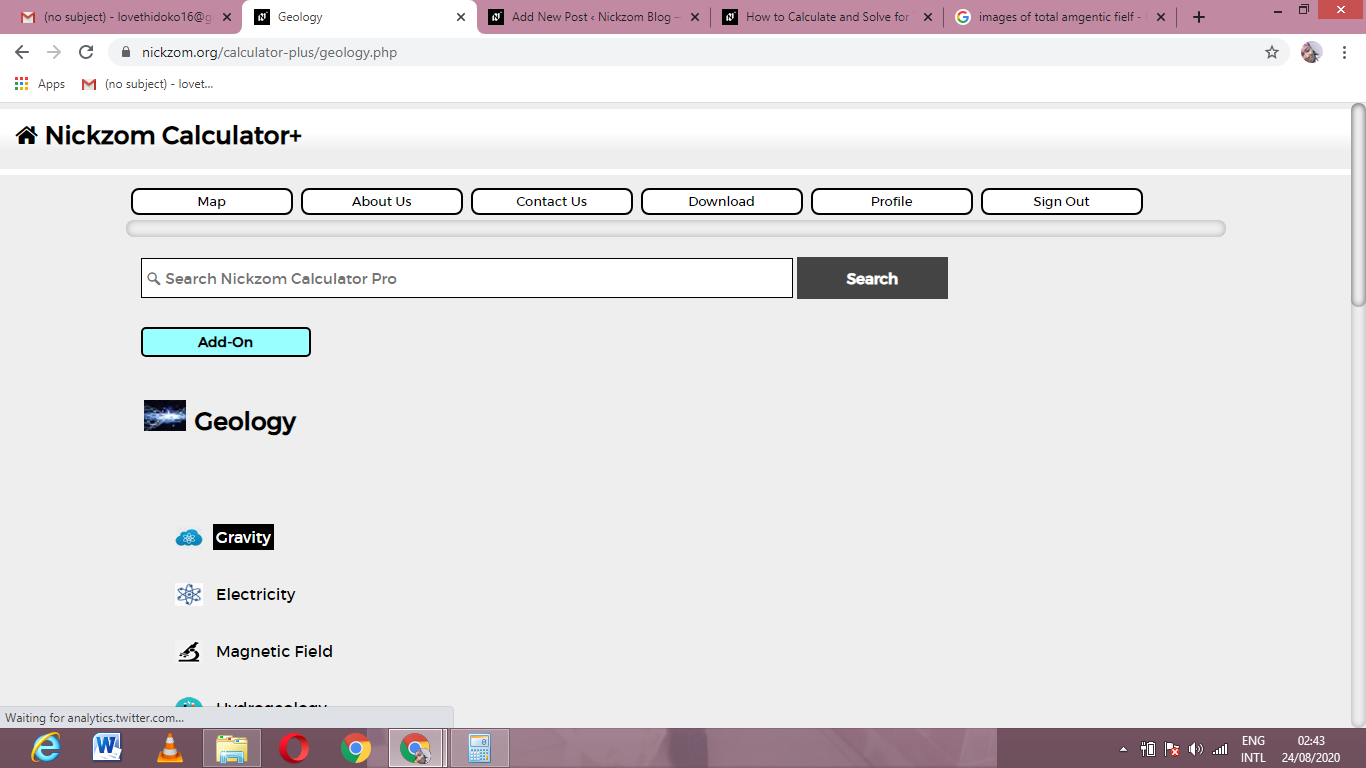Now, Click on Actual Mass of Geological Body under Gravity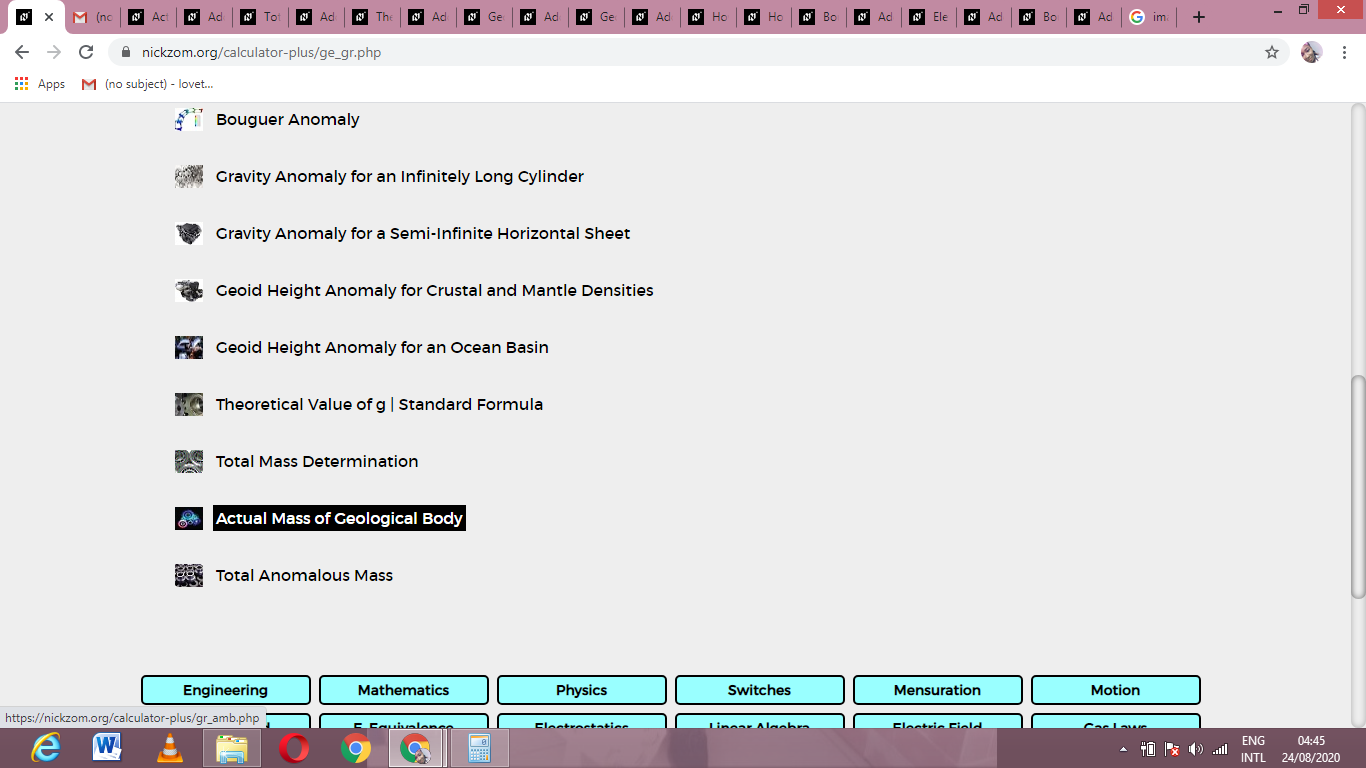The screenshot below displays the page or activity to enter your values, to get the answer for the actual mass of geological body according to the respective parameters which are the Total Anomalous Mass (ME), Anomalous Density of the Body (ρ1and Host Rock Density (ρ0).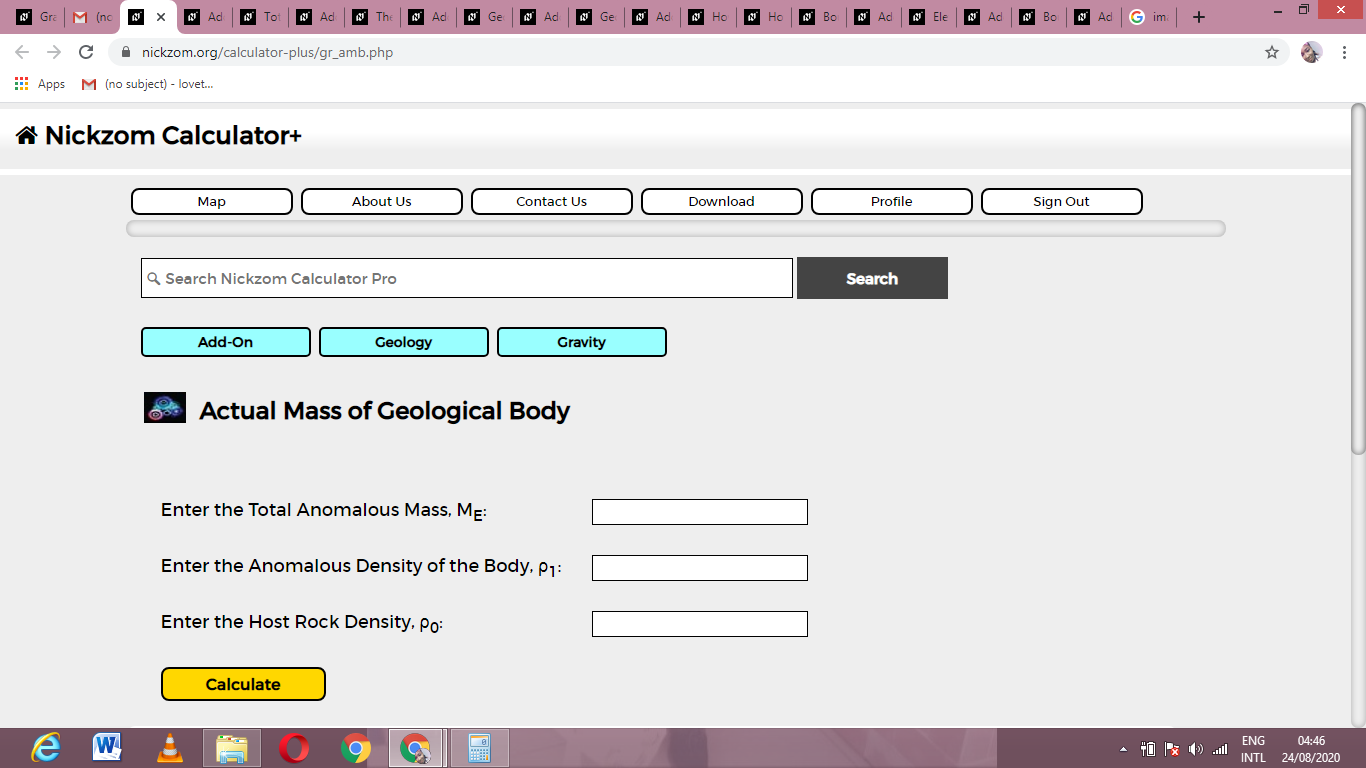Now, enter the values appropriately and accordingly for the parameters as required by the Total Anomalous Mass (ME) is 12, Anomalous Density of the Body (ρ1is 21 and Host Rock Density (ρ0) is 13.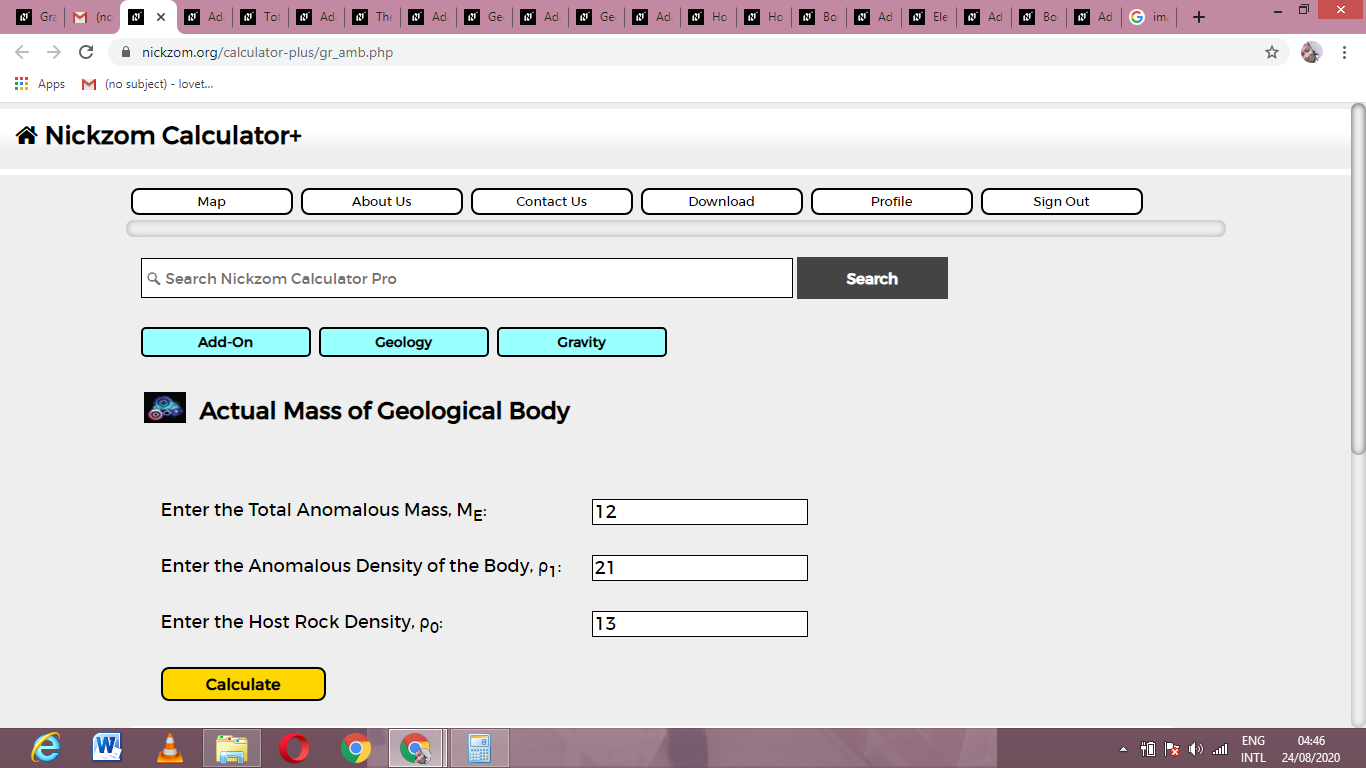Finally, Click on Calculate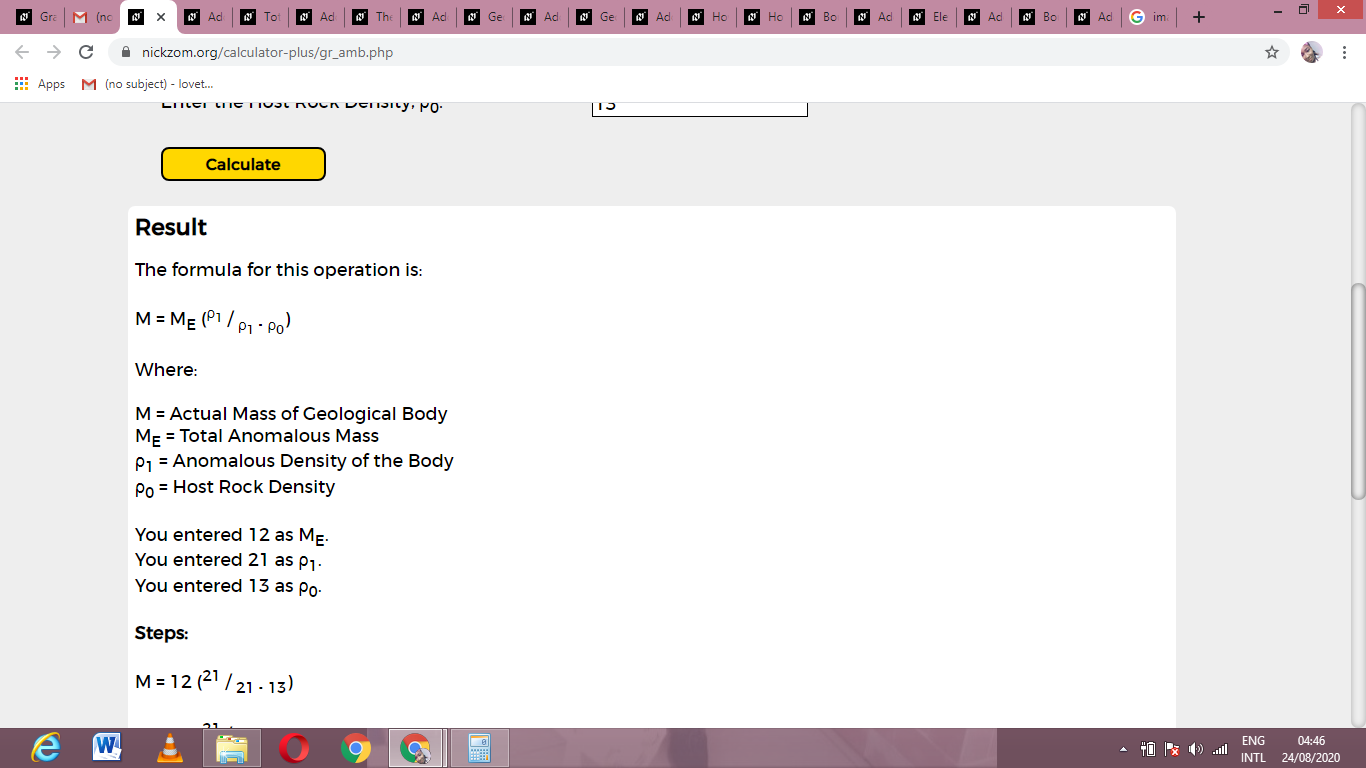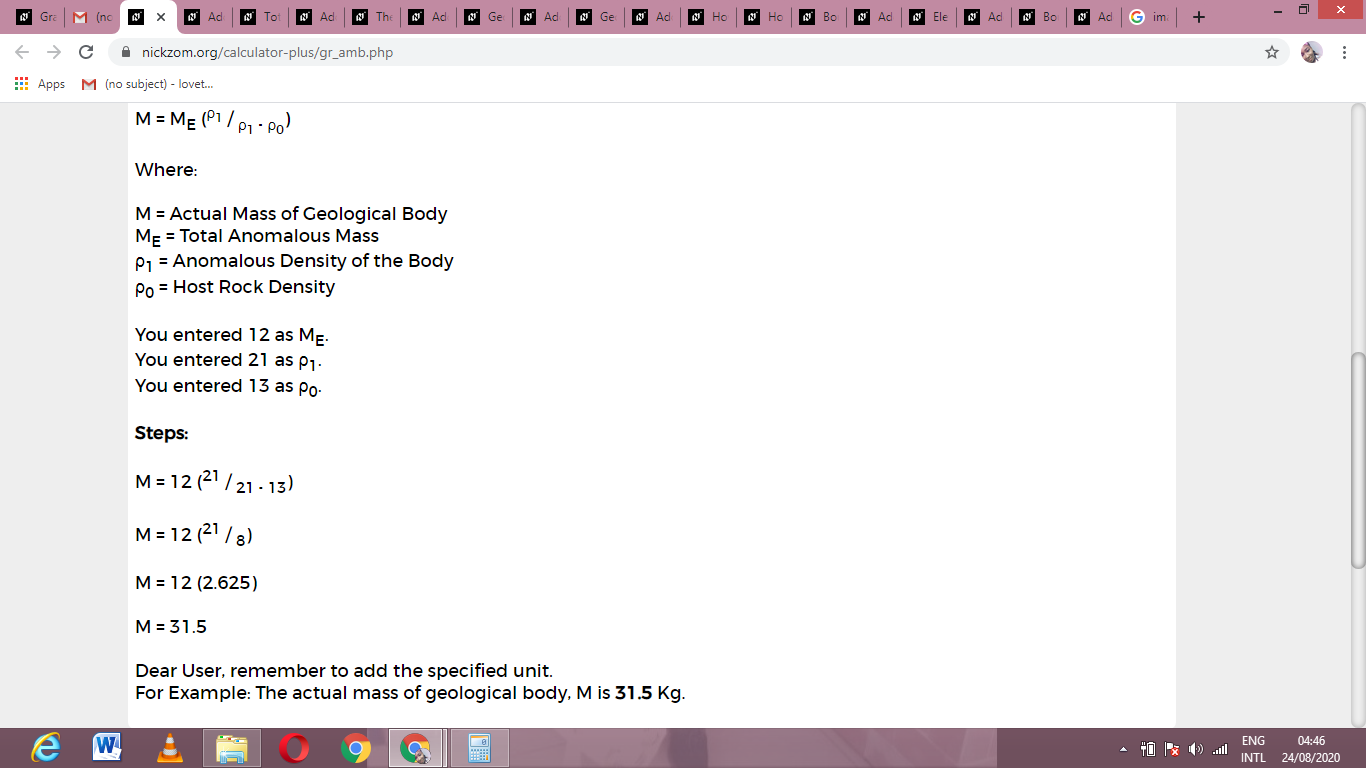As you can see from the screenshot above, Nickzom Calculator– The Calculator Encyclopedia solves for the actual mass of geological body and presents the formula, workings and steps too.

## 2 thoughts on “How to Calculate and Solve for Actual Mass of Geological Body | Gravity”

1.Parbriz Otokar Navigo 2006 says:

I blog quite often and I seriously appreciate your information. This article has truly peaked
my interest. I am going to take a note of your site and keep checking for
new details about once a week. I subscribed to your Feed too. https://anunturi-parbrize.ro/parbriz-otokar-51.html

1.Loveth Idoko says:

thank you.